nextnano.com  nextnano³  Download | Search | Copyright | Publications  * password protected nextnano³ software3D pyramidal QD

# nextnano3 - Tutorial

## Energy levels in a pyramidal shaped InAs/GaAs quantum dot including strain and piezoelectric fields

Author: Stefan Birner

```-> 3DInAsGaAsQDPyramid_PryorPRB1998_10nm_nn3.in / *nnp.in - ```input file for the nextnano3 and nextnano++ software

## Energy levels in a pyramidal shaped InAs/GaAs quantum dot including strain and piezoelectric fields

```-> 3DInAsGaAsQDPyramid_PryorPRB1998_10nm.in  - ```QD with 10 nm base length

This tutorial is based on the following paper:

Eight-band calculations of strained InAs/GaAs quantum dots compared with one-, four-, and six-band approximations
Craig Pyror
Physical Review B 57 (12), 7190 (1998)

We use identical material parameters with respect to this paper in order to make it possible to reproduce Pryor's results.

We note that meanwhile more realistic material parameters are available and that for the simulation of realistic quantum dots the inclusion of the wetting layer and an appropriate nonlinear InGaAs alloy profile is recommended.

We make the following simplifications in order to be consistent with Pryor:

• The wetting layer is omitted for simplicity.
• The QD material is purely InAs.
• The barrier material is purely GaAs.
• The dielectric constant in the barrier material (GaAs) is the one for InAs.
• Periodic boundary conditions are assumed in all three directions for the strain equation.
• The QD shape is a pyramid with a square base (base length = 10 nm) and a height of 5 nm.
The four side walls of the pyramid are oriented in the (011), (0-11), (101) and (-101) planes, respectively.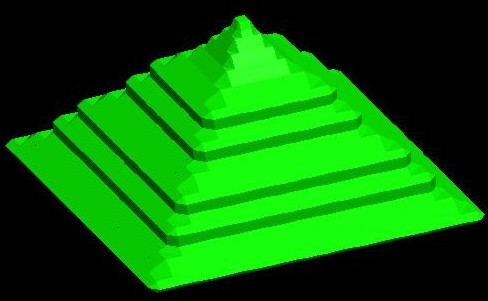The whole simulation area has the dimensions 44 nm x 44 nm x 40 nm.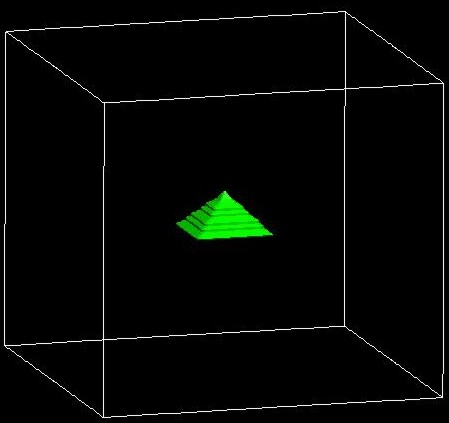### Conduction and valence band profiles

The following figures shows the conduction and valence band edges (heavy hole, light hole and split-off hole) for a 10 nm pyramidal shaped QD along two different line scans.
The energies of the bands have been obtained by diagonalizing the 8-band k.p Hamiltonian at k = 0 (including the Bir-Pikus strain Hamiltonian) for each grid point, taking into account the local strain tensor and deformation potentials.
Note that piezoelectric effects are not included yet in this band profile.

The left   figure shows the band profile along the z axis through the center of the QD (x = y = 0 nm).
The right figure shows the band profile along the x axis through the base   of the QD (y = z = 0 nm).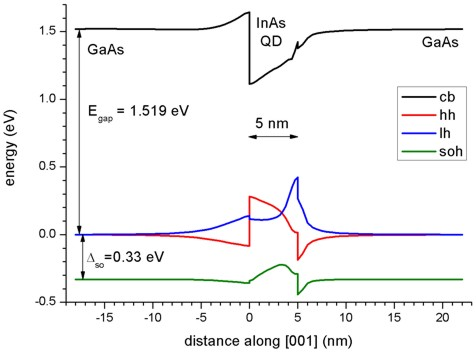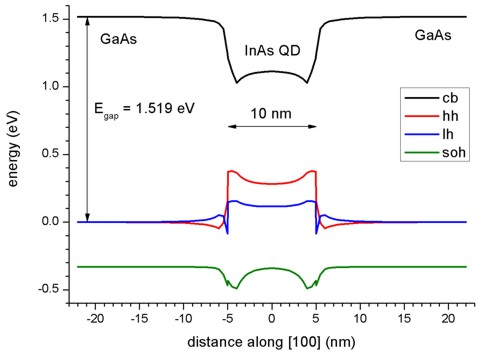The figures compare well with Pryor's Figs. 2(a) and 2(b). However, there are some differences:
Due to valence band mixing of the states in the k.p Hamiltonian, we do not have pure heavy and light hole eigenstates any more.
Thus there is some arbitrariness to assign the labels "heavy" and "light" to the relevant eigenstates h1 and h2.
Obviously, when solving the full 6-band or 8-band k.p Hamiltonian, this labelling becomes irrelevant because all three hole band edges enter the Hamiltonian simultaneously (in contrast to a single-band effective mass approach where only individual "heavy" hole or "light" hole band edges would be considered).

### Electron wave function of the ground state (single-band effective-mass approximation)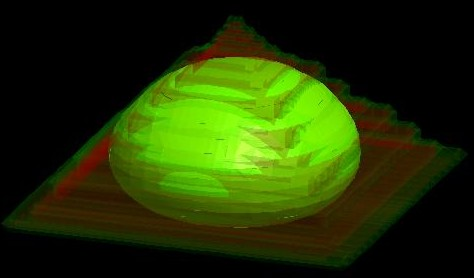... to be continued. Please use the nextnano++ software for Quantum Dots (`3DInAsGaAsQDPyramid_PryorPRB1998_10nm_nnp.in`). It is much faster.

Preliminary:

10 nm quantum dot (Note: Pryor's Fig. 7 shows the energies for a 14 nm quantum dot).

band gap: 1.519 eV

Electron energies
(i)   effective mass (me = 0.023 m0)``` => 0.7000983 ```eV (only one confined electron state)
(ii)  effective mass (me = 0.04   m0)``` => ``` eV
(iii) effective mass (me(r) = ...   m0)``` => ```not implemented in nextnano³
(iv) 8-band k.p```                => ``` eV

Hole energies
( )   effective mass (mhh = 0.41 m0)``` => hh1 = -0.585198481``` eV
```                           => hh1 = -0.61776``` eV
```                           => hh1 = -0.62275``` eV
(i)   6-band k.p```                => 1.0081402 ```eV (?) (bad eigenvalues using 6-band k.p with``` finite-differences```)
(ii)  8-band k.p```                => ``` eV

Transition energy electron - hole:
- (i) - ( ): exciton correction 2.9 meV (Pryor: 27 meV)
```  E_ex [eV]   E_el - E_hl   E_el0 - E_hl0   Delta_Ex     REAL(inter_matV(1))   1.28238     1.27958       1.28530         0.00291947   0.428169  ```

14 nm quantum dot (Pryor's Fig. 7).

Electron energies
(i)   effective mass (me = 0.023 m0)``` => 0.6458949``` eV (only one confined electron state) + (1.519 - 0.752916) eV = 1.412 eV (in substrate layer below QD)
(i)   effective mass (me = 0.023 m0)``` => 0.6458949``` eV (only one confined electron state) + (1.519 - 0.765522) eV = 1.399 eV (in substrate layer at corner)
(i)   effective mass (me = 0.04   m0)``` => 0.6248762``` eV (only one confined electron state) + (1.519 - 0.765522) eV = 1.378 eV (in substrate layer at corner)

14 nm, 6x6k.p, box, nonsym:
-0.56607270
-0.58734305
-0.59621434
-0.60757551
-0.62802221
-0.63650764